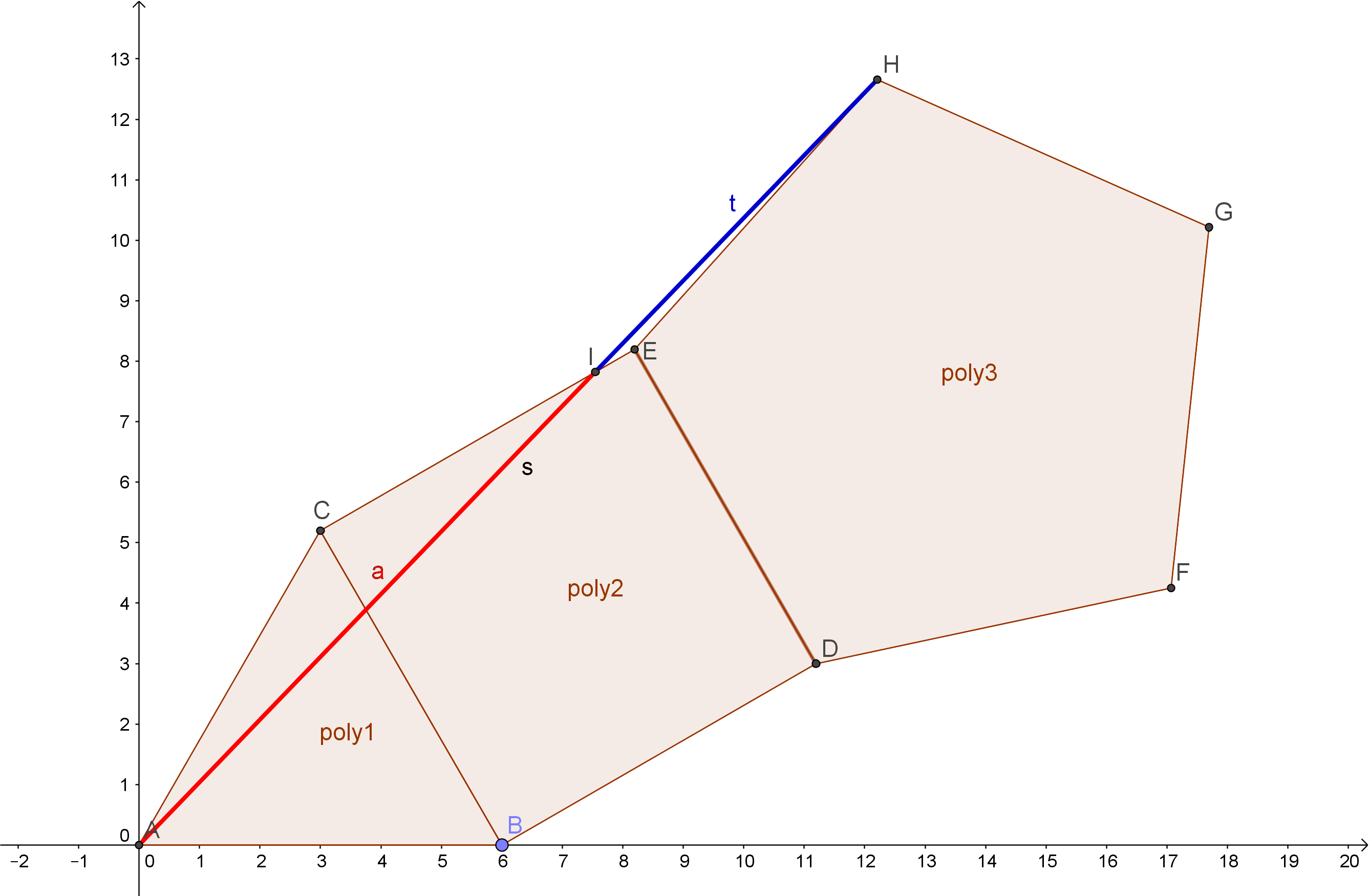# IncompletenessMathematical Surrealism

I’m a system
of strict and rigorous rules.
Don’t dare to break them
or you are incredible fools!

Prove me wrong,
but don’t dare
to leave me along
for it’s all we ever were.

Don’t dare to leave me,
because I’m all of this,
don’t dare to be
that very last beautiful kiss.

But what are you doing?
Where are you going
after all?
What were you saying in your very last call?

Are you telling me
I’m incomplete,
that no one can see
me being utterly complete?

What am I supposed to be
without a complete identity?
being self-referent is all I can!

View original postMathematical Surrealism

If I’m true and false at the same time
yet it’s a tautology in turn.

So what if I’m
true or false
and false and true?

What if I’m red and blue,
but not pink
in turn?

If I’m a symbiosis
of one and zero,
why am I not an in-between?

Superposed, I am,
that’s all
I truly am!

View original post

# The Mathemagician’s RoomIsles of Time

It’s a place full of wonder, strangeness and bizarre beauty: the room – or should I say space – of a “mathematician that’s merely a magician.

It’s a common optical illusion, a kind of tricky paradox. “If our brains are simple enough for us to understand them, then we’d be so simple that we couldn’t.” ~ Quote by Ian Steward (a mathematician)

The floor itself is an impossible figure – a typical symbol for paradoxes…
The spirals on the checkerboard-floor are Fibonacci spirals.

This drawing is mostly about the beauty of Phi Φ, Psi ψ and the Fibonacci-sequence.

The fibonacci sequence
The fibonacci sequence works this way:
1+1 = 2
1+2 = 3
2+3 = 5
3+5 = 8
5+8 = 13
8+13 = 21
13+21 = 34
21+34 = 55
34+55 = 89
55+89…
… and so on…
The sequence then is: 1;1;2;3;5;8;13;21;34;55;89;…….

Let’s play with these numbers!
1/1=1
2/1=2
3/2=1.5

View original post 305 more words

# Platonic Solids and the Golden Ratio

There are five regular polyhedra. These are they: tetrahedron, cube/hexahedron, octahedron, dodecahedron and icosahedron. Here is a short summary in form of a table about the vertices, edges, faces and volumes of these solids:

 Tetrahedron Cube/ Hexahedron Octahedron Dodecahedron Icosahedron v vertices 4 8 6 20 12 e edges 6 12 12 30 30 f faces 4 6 8 12 20 V volume √2 /12*a³ a³ √2 /3*a³ (15+7*√5)/4*a³ (15+5*√5)/12*a³

We could group them into three groups:

• The triangular faced ones {tetrahedron, octahedron, icosahedron}

• The square faced one {cube}

• The pentagon faced one {dodecahedron}

If we put these polygons together like this, we can recognize the golden ratio in it: Here we add a triangle, square and a pentagon together in the following way. Then we connect the point A and H with each other – and exactly where this line crosses the square the golden ratio appears:Source
Also, if you didn’t know about the pentagram, even there appears the golden ratio:Source: Wikipedia

And, two of the platonic solids have the golden ratio in themselves: the icosahderon and the dodecahedron.

Imagine 3 planes put together like this:In a dodechedron the exact same planes can be put into it like this:But if we want the edges of the planes to be on the edges of the dodecahedron, we have to use another ratio:Then the ratio is 1 to phi².